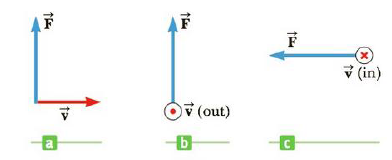Chapter 19, Problem 3P

Chapter
Section
Textbook Problem

Find the direction of the magnetic field acting on the positively charged particle moving in the various situations shown in Figure P19.3 if the direction of the magnetic force acting on it is as indicated.Figure P19.3 (Problems 3 and 25) For Problem 25, replace the velocity vector with a current in that direction.

a)

To determine
The direction of the magnetic field.

Explanation

Given info: The velocity of the positively charged particle is directed rightward on the plane of the paper. The magnetic force is directed on the plane of the paper and pointing upward.

Explanation:

Right hand rule: When the fingers of the right hand are placed pointing the direction of the velocity of the positively charged particle, the thumb p...

b)

To determine
The direction of the magnetic field.

c)

To determine
The direction of the magnetic field.

Still sussing out bartleby?

Check out a sample textbook solution.

See a sample solution

The Solution to Your Study Problems

Bartleby provides explanations to thousands of textbook problems written by our experts, many with advanced degrees!

Get Started